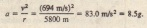# PROBLEM SOLVING TACTICS

PROBLEM SOLVING TACTICS

Tactic 1: Numbers Versus Algebra

One way to avoid rounding errors and other numerical errors is to  solve problems algebraically, substituting numbers only in the final step. That is easy  and that is the way experienced problem solvers operate. In these early chapters. however. we prefer to solve most problems in pans. to give you a firmer numerical grasp of what is going on. Later we shall stick to the algebra longer.

Uniform Circular Motion

A particle is in uniform circular motion if it travels around a circle or a circular arc at constant (uniform) speed. Although the speed does not vary. the particle is accelerating.That fact may be surprising because we often think of acceleration (a change in velocity) as an increase or decrease in speed. However. actually velocity is a vector. not a scalar. Then even if a velocity changes only in direction. there is still an acceleration. and that is what happens in uniform circular motion.Velocity and acceleration vectorsfor a panicle in counterclockwiseuniform circular motion. Both haveconstant magnitude but vary continuouslyin direction

Proof

To find the magnitude and direction of the acceleration for uniform circular motion, we consider particle p moves at constant speed v around a circle of radius r. At the instant shown, p has coordinates x and y.Now note that the rate changes is equal to the velocity component.Similarly we see that v. Making these substitutions in. We findThis vector and its components.Following  we find that the magnitude.Particle moves in counterclockwiseuniformcircular motion.(a) Its position and velocity Vat a certaininstant. (b) Velocity Vand itscomponents. (e) The particle’s accelerationa and its components

CHECKPOINT 6: An object moves at constant speed along a circular path in a horizontal. problem,with the center at the origin. When the object is at x velocity. Give the object’s (a) velocity and (b) acceleration when it is at).

Sample Problem
Top gun pilots have long worried about taking a turn too tightly. As a pilot’s body under goes centripetal acceleration, with the head toward the center , the blood pressure in the brain decreases, leading to loss of brain function.

There are several warning signs to signal a pilot to ease up  when the centripetal acceleration is 2 or 3. the pilot feels heavy. At about 4, the pilot’s vision switches to black and white and narrows to “tunnel vision.” If that acceleration is sustained.SOLUTION: The Key idea here is that although the pilot’s speed is constant. the circular path requites a (centripetal) acceleration with.If an unwary pilot caught in a dogfight puts the aircraft into such a tight turn. the pilot goes  almost immediately. with no warningsigns to signal the danger